# Root system

A finite set $R$ of vectors in a vector space $V$ over $\mathbf R$, possessing the following properties: 1) $R$ does not contain the null vector, and it generates $V$; 2) for every $\alpha \in R$ there exists an element $\alpha ^{*}$ of the space $V ^{*}$ dual to $V$ such that $\alpha ^{*} (\alpha) =2$ and such that the endomorphism $s _ \alpha : \ x \mapsto x-\alpha ^{*} (x) \alpha$ of $V$ maps $R$ into itself; 3) $n ( \alpha ,\ \beta ) = \beta ^{*} ( \alpha ) \in \mathbf Z$ for all $\alpha ,\ \beta \in R$.

Sets of vectors with the properties listed above first arose in the theory of semi-simple complex Lie algebras, as weight systems of the adjoint representation of a maximal torus in such an algebra (see Weight of a representation of a Lie algebra; Lie algebra, semi-simple). Later it was noticed that such systems of vectors appear naturally in many other branches of mathematics, such as algebraic geometry , , the theory of singularities  and the theory of integer-valued quadratic forms . Certain problems in number theory have also turned out to be connected with root systems .

## General properties of root systems.

The endomorphism $s _ \alpha$ is a reflection relative to $\alpha$ and is uniquely determined by the properties 1) and 2). The set of fixed points of $s _ \alpha$ is $\mathop{\rm Ker}\nolimits \ \alpha ^{*}$, and $s _ \alpha ( \alpha ) =-\alpha$. The elements of $R$ are called the roots of the root system $R$. Its rank is $\mathop{\rm dim}\nolimits \ V$. A root system $R$ is said to be reduced if, for any $\alpha \in R$, the vector $-\alpha$ is the only root collinear with $\alpha$. The set $R ^{*} = \{ {\alpha ^ *} : {\alpha \in R} \}$ is a root system in $V ^{*}$, and $\alpha ^{**} = \alpha$ for all $\alpha \in R$; it is called the dual (or inverse) of $R$. The finite group $A (R)$ generated by all automorphisms of $V$ which map $R$ onto itself is called the automorphism group of the root system $R$. The subgroup $W (R)$ of $A (R)$ generated by the reflections $s _ \alpha$, $\alpha \in R$, is called the Weyl group of $R$. If $V$ is a direct sum of subspaces $V _{i}$, $i = 1 \dots l$, and $R _{i}$ is a root system in $V _{i}$, then $R = \cup _ {i = 1} ^{l} R _{i}$ is a root system in $V$, called the direct sum of the root systems $R _{i}$. A non-empty root system $R$ is said to be irreducible if it is not the direct sum of two non-empty root systems. Every root systems is the direct sum of some set of irreducible root systems, and this decomposition is unique up to the order of terms.

The connected components of the set $V-\cup _ {\alpha \in R} \mathop{\rm Ker}\nolimits \ \alpha ^{*}$ are open simplicial cones, known as the chambers of the root system $R$ in $V$( see Chamber). The Weyl group acts in a simply transitive manner on the set of all chambers. The closure $\overline{C}$ of any chamber $C$ is a fundamental domain of the discrete group $W (R)$. Let $L _{1} \dots L _{r}$ be the walls of a chamber $C$. For each wall $L _{i}$ there exists a unique root $\alpha _{i}$ such that $L _{i} = \mathop{\rm Ker}\nolimits \ \alpha _{i} ^{*}$ and $\alpha _{i}$ lies on the same side of $L _{i}$ as $C$. The family of roots $\alpha _{1} \dots \alpha _{r}$ forms a basis in $V$, called the basis of the root system defined by the chamber $C$. One also says that $\alpha _{1} \dots \alpha _{r}$ is the set of simple roots defined by $C$. The group $W (R)$ is generated by the reflections $s _ {\alpha _ i}$, $i = 1 \dots r$, and moreover $(s _ {\alpha _ i} s _ {\alpha _ j} ) ^ {m _ ij} = 1$, where $m _{ij}$ is the order of $s _ {\alpha _ i} s _ {\alpha _ j}$, is a system of defining relations for $W (R)$, so that $W (R)$ is a Coxeter group. The group $A (R)$ is the semi-direct product of the subgroup of all elements in $A (R)$ that leave the set $\alpha _{1} \dots \alpha _{r}$ invariant, with $W (R)$.

A choice of a chamber $C$ defines an order relation on $V$( compatible with the vector-space structure), with respect to which the positive elements are precisely the linear combinations of the simple roots $\alpha _{1} \dots \alpha _{r}$ with non-negative coefficients. Any root is either positive or negative, and all its coordinates with respect to the basis $\alpha _{1} \dots \alpha _{r}$ are integers. The subgroup $Q (R)$ of $V$ generated by the root system $R$ is a lattice (i.e. a discrete subgroup of rank $r$, cf. Lattice in a Lie group) invariant under the Weyl group $W (R)$. Its elements are called the radical weights of the root system $R$. The Weyl groups of root systems are precisely those discrete linear groups generated by reflections that leave no non-zero vectors fixed and possess an invariant lattice. If $Q (R)$ is regarded as a group of translations of the space $V$, then the semi-direct product $W _{a} (R)$ of $W (R)$ and $Q (R)$ is known as the affine Weyl group of $R$. $W _{a} (R)$ is a discrete group of transformations of $V$ generated by reflections in the hyperplanes $$L _ {\alpha , k} = \{ {v \in V} : {\alpha ^{*} (v) = k} \} ,$$ where $\alpha \in R$, $k \in \mathbf Z$. The quotient space of $V$ by $W _{a} (R)$ is compact; if $R$ is irreducible, then a fundamental domain for $W _{a} (R)$ is a simplex.

One can choose a positive-definite symmetric bilinear form $( \ ,\ )$ on $V$ which is invariant under $W (R)$( the choice is not unique). This form gives $V$ the structure of a Euclidean space in which the elements of $W (R)$ are orthogonal transformations, while the reflections $s _ \alpha$, $\alpha \in R$, have the following form for all $x \in V$: $$s _ \alpha (x) = x-2 (x,\ \alpha ) \frac \alpha {( \alpha ,\ \alpha )} .$$ Using the form $( \ ,\ )$, one can identify the spaces $V$ and $V ^{*}$, and then $\alpha ^{*} = 2 \alpha /( \alpha ,\ \alpha )$; condition 3) in the definition of a root system means then that $n ( \alpha ,\ \beta ) = 2 ( \alpha ,\ \beta )/ ( \beta ,\ \beta ) \in \mathbf Z$ for all $\alpha ,\ \beta \in \mathbf R$.

Thanks to the form $( \ ,\ )$ one can speak of metric relations between roots, in particular of the angle between roots and of the length of a root. It turns out that the magnitude of the angle is independent of the choice of $( \ ,\ )$, while if a root system $R$ is irreducible, then this is also true for the ratio of the lengths of two roots.

## Classification of root systems.

Let $\alpha _{1} \dots \alpha _{r}$ be some fixed basis of a reduced root system $R$ and let $n _{ij} = n ( \alpha _{i} ,\ \alpha _{j} )$. The matrix $\| n _{ij} \|$, $1 \leq i,\ j \leq r$, is called the Cartan matrix of the root system $R$; this matrix has $n _{ii} = 2$, and $n _{ij}$( $i \neq j$) may be $0,\ -1,\ -2$, or $-3$. Up to permutation of indices, the Cartan matrix is independent of the choice of the basis. Two root systems with identical Cartan matrices are isomorphic.

With any root system one usually associates its Coxeter graph, the vertices of which are the basis elements $\alpha _{1} \dots \alpha _{r}$, with vertices $\alpha _{i}$ and $\alpha _{j}$ joined by one, two or three edges or not joined at all, respectively, according as the product $n _{ij} n _{ji}$ equals $1,\ 2,\ 3$, or $0$. A root system is irreducible if and only if its Coxeter graph is connected. The Coxeter graph defines only the angles between pairs of basic roots; it does not determine the Cartan matrix (though it does determine the Weyl group): There exist dual non-isomorphic root systems with the same Coxeter graph. However, the Cartan matrix (and together with it the root system) is completely determined by the directed Coxeter graph, also known as the Dynkin diagram or simple root diagram of the root system. The direction is defined by the rule: If the simple roots $\alpha _{i}$ and $\alpha _{j}$ are not orthogonal and are different in length, the two or three edges joining the $i$- th and $j$- th vertices are assigned the inequality sign $>$, directed towards the vertex corresponding to the root of smaller length. In some cases, above each vertex of the Coxeter graph one writes a number proportional to the squared length of the corresponding root (the same proportionality factor for all the roots); this weighted graph also uniquely determines the original root system.

The following is a complete list of the pairwise non-isomorphic, irreducible, reduced root systems, as defined by their simple root diagrams: vertices vertices vertices vertices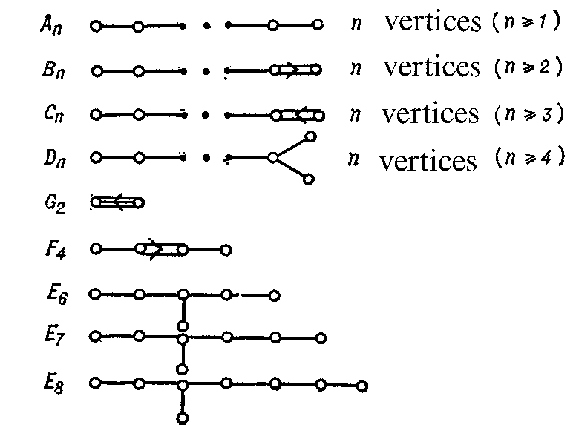Figure: r082590a

## Construction of the irreducible root systems.

Let $e _{1} \dots e _{n}$ be the canonical basis in $\mathbf R ^{n}$, $( \ ,\ )$ the standard scalar product in $\mathbf R ^{n}$ for which $(e _{i} ,\ e _{j} ) = \delta _{ij}$, and $\Gamma _{n}$ the lattice in $\mathbf R ^{n}$ generated by the vectors $e _{1} \dots e _{n}$.

1) Let $V$ be a hyperplane in $\mathbf R ^ {n + 1}$ orthogonal to the vector $e _{1} + \dots + e _ {n + 1}$. Then $$R = \{ {\alpha \in V \cap \Gamma _ {n + 1}} : { ( \alpha ,\ \alpha ) = 2} \} =$$ $$= \{ {e _{i} -e _ j} : {i \neq j, i,\ j = 1 \dots n + 1} \}$$ is a root system of type $A _{n}$. For $n = 2$, this root system has the form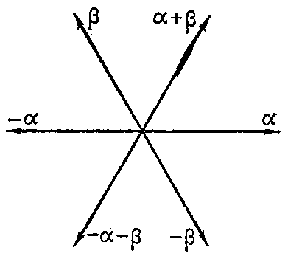Figure: r082590b

2) The set of vectors $$\{ {\alpha \in \Gamma _ n} : {( \alpha ,\ \alpha ) = 1 \textrm{ or } 2} \} =$$ $$= \{ {\pm e _{i} , \pm e _{i} \pm e _ j} : {i\neq j, i,\ j = 1 \dots n} \}$$ in $\mathbf R ^{n}$ is a root system of type $B _{n}$. For $n = 2$ it has the form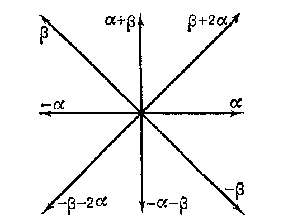Figure: r082590c

3) A root system of type $C _{n}$ is dual to a system of type $B _{n}$ and consists of the vectors $$\{ {\pm e _{i} \pm e _{j} , \pm 2e _ i} : {i \neq j, i,\ j = 1 \dots n} \} .$$ 4) The set of vectors $$\{ {\alpha \in \Gamma _ n} : { ( \alpha ,\ \alpha ) = 2} \} = \{ {\pm e _{i} \pm e _ j} : { i \neq j, i,\ j = 1 \dots n} \}$$ in $\mathbf R ^{n}$ is a root system of type $D _{n}$.

5) A root system of type $G _{2}$ has the form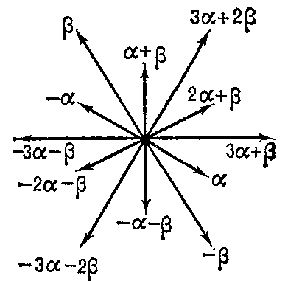Figure: r082590d

and it can be described as the set of algebraic integers of the cyclotomic field generated by a cubic root of unity with norm $1$ or $3$.

6) The set of vectors $$\left \{ {\pm e _{i} , \pm e _{i} \pm e _{j} , { \frac{1}{2} } ( \pm e _{1} \pm e _{2} \pm e _{3} \pm e _{4} ) } : {i \neq j, i,\ j = 1 \dots 4} \right \}$$ in $\mathbf R ^{4}$ is a root system of type $F _{4}$.

7) The set of vectors $$\left \{ {\pm e _{i} \pm e _{j} , { \frac{1}{2} } \sum _ {i = 1} ^ 8 (-1) ^ {m (i)} e _ i} : {i \neq j, i,\ j = 1 \dots 8} \textrm{ with } {\sum_{i=1}^8 m (i) \equiv 0 \mathop{\rm mod}\nolimits \ 2} \right \}$$ in $\mathbf R ^{8}$ is a root system of type $E _{8}$.

8) A root system of type $E _{6}$ can be obtained as the intersection of a root system of type $E _{8}$ with the subspace of $\mathbf R ^{8}$ spanned by $e _{1} \dots e _{6}$.

9) A root system of type $E _{7}$ can be obtained as the intersection of a root system of type $E _{8}$ with the subspace of $\mathbf R ^{8}$ spanned by $e _{1} \dots e _{7}$.

10) For each dimension $n \geq 1$ there exists (up to an isomorphism) exactly one non-reduced irreducible root system $BC _{n}$, namely, the union of $B _{n}$ and $C _{n}$( see above). For $n = 2$ the system has the form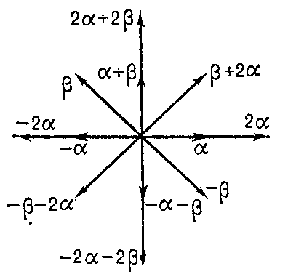Figure: r082590e

Concerning affine root systems, see .

How to Cite This Entry:
Root system. Encyclopedia of Mathematics. URL: http://encyclopediaofmath.org/index.php?title=Root_system&oldid=44314
This article was adapted from an original article by V.L. Popov (originator), which appeared in Encyclopedia of Mathematics - ISBN 1402006098. See original article